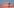# To float, or not to float – Choosing correct numeric data type in Snowflake

Fixed-point numerical data types should be the default choice when designing Snowflake relational tables. Using floating-point data types has multiple downsides, which are important to understand. The effect of choosing different numerical data type can be easily tested.

Snowflake numeric data types can be split in two main categories: fixed-point numbers and floating-point numbers. In this blog post we are going to look at what these mean from Snowflake database design point of view, and especially should you use floating type when dealing with numeric data?

Fixed-point numbers are exact numeric values, which include data types such as number, integer and decimal. For these data types, developer can determine precision (allowed number of digits in column) and scale (number of digits right of the decimal point). In Snowflake, all fixed-point numeric data types are actually type decimal with precision 38 and scale 0, if not specified differently. Typical use cases for fixed-point data types are natural numbers and exact decimal values, such as monetary figures, where they need to be stored precisely.

On the other side of the spectrum are floating-point numbers, which are approximate representations of numeric values. In Snowflake, floating-point columns can be created with key-words float, double, double precision or real. However, as the Snowflake documentation states, all of these data types are actually stored as double and they do not have difference in precision, but displayed as floats. Floating-point data types are mainly used in mathematics and science to simplify the calculations with scientific notation. Storing numbers with major differences in magnitude is their advantage in databases too, because zeros trailing or following the decimal sign does not consume memory as it does for decimal format. In traditional computing, floats are considered faster for computation, but is that really the case in modern database design?

## Floating-point precision

First, let us explore inserting data on Snowflake into table with float as numeric data type. We create a table and insert 500 million rows of generated dummy data to the table with following query:

```CREATE TABLE T_FLOAT_500M (
id            INT,
float_field   FLOAT );

INSERT INTO T_FLOAT_500M
SELECT SEQ8()
, UNIFORM(1, 500000000, RANDOM())::FLOAT
FROM TABLE ( GENERATOR ( ROWCOUNT => 500000000 ) );```

To see the effect of using float as the data type for big numeric values, we can run the following query:

```SELECT SUM(float_field) AS NO_CONVERSION
, SUM(float_field::INT) AS CONVERT_VALUES_SEPARATELY
, SUM(float_field)::INT AS CONVERT_SUM_VALUE
FROM T_FLOAT_500M;```

Sum without the conversion produces us a rounded number with scientific notation: 1.24997318579082e+17
Separately converted values sum produces result: 124997318579081654
Conversion made after the calculation produces sum value: 124997318579081664

From the sum results, we will notice the accuracy problem related to storing numeric values as floats as the sum results differ from each other. When dealing with large or extremely accurate numeric values, floats may cause differentiation in results due to their nature of being approximate representations. Same effect can be seen when using WHERE clauses as the approximate representations may not work as designed with conditions that point to exact numeric values.

## Storage size of float

Next, we create a similar table as earlier, but with the second field being type integer and populate it without converting random figures to floats.

```CREATE TABLE T_INT_500M (
id          INT,
int_field   INT);

INSERT INTO T_INT_500M
SELECT SEQ8()
, UNIFORM(1, 500000000, RANDOM())::INT
FROM TABLE ( GENERATOR ( ROWCOUNT => 500000000 ) );

SHOW TABLES LIKE '%_500M';```

Looking at the Snowflake table statistics, we will notice integer table is smaller (3.37 GB) compared to the float table (5.50 GB). The difference in table sizes is significant with the float table being 63 % bigger. This can be explained by Snowflake reserving 64 bits of memory for every float value. Integer values on the other hand are stored in compressed format and take only the necessary amount of memory.

This difference is seen also on SELECT queries, where querying all rows with X-Small warehouse takes only 85 seconds for integer type table compared to the 160 seconds with the float type table. Difference is once again major.

## Summary

Floats still have their use cases with numbers that have majorly different magnitudes. But from the presented test cases, we can draw a conclusion that using floating-point data types will lead to bigger storage sizes and longer query times, which result as an increase to data warehousing costs. Another thing to consider is the possibility of imprecise values, when dealing with extremely accurate data. Whenever possible, it is recommended not to use float as a type for numeric data without a specific reason. Especially precise and whole numbers are not meant to be stored as floats and should be given appropriate data types.